Vol. LX, No. 10, December 1953

AN EVERYWHERE CONTINUOUS NOWHERE DIFFRENTIABLE FUNCTION

John McCarthy, Princeton University

The following is an especially simple example. It is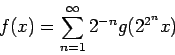where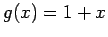for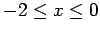,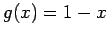for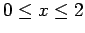and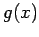has period 4.

The function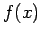is continuous because it is the uniform limit of continuous functions. To show that it is not differentiable, take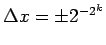, choosing whichever sign makes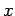and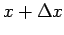be on the same linear segment of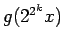. We have

1.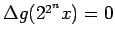for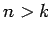, since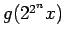has period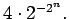2.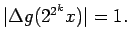3.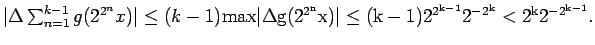Hence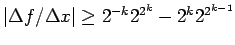which goes to infinity with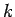.

The proof that the present example has the required property is simpler than that for any other example the author has seen.

Weierstrass gave the example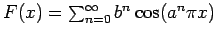for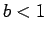and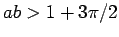which is discussed in Goursat-Hedrick Mathematical Analysis.

A complete discussion of functions with various singular properties is given in Hobson, Functions of a Real Variable, volume II, Cambridge, 1926.

2006 January note: I was tempted to dig up this 1953 note of mine and put it on my web page by reading The Calculus Gallery by William Dunham. This excellent book includes the first proofs of a number of important theorems, including Weierstrass's proof that his function has the required properties. Dunham's version of Weierstrass's proof is six pages of what Dunham describes as difficult mathematics. Since my proof is 13 lines of what I consider easy math, I decided to copy my old note and discuss it. Dunham recounts that the famous mathematicians Hermite, Poincare and Picard all expressed themselves as repelled by Weierstrass's pathological example''. I'm sure that by the time I was born in 1927, such functions were no longer regarded as repellent. My own opinion is that most everywhere continuous functions, in some suitable sense of most, are nowhere differentiable.

Remarks:

1. To prove a function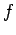differentiable at, one must show that no matter how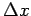goes to zero,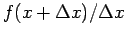approaches a limit. To provenon-differentiable at, one need only find a sequence of values offor which the limit doesn't exist.

2. Ifis to be represented as the sum of a series of continuous functions, it suffices to bound the terms by a suitable positive terms,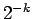in our case. Thenis sure to be everywhere continuous. It doesn't matter how fast the successive terms wiggle.

3. In our case, the terms are. The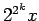grows fast enough to overcome thedamping.

4. I would expect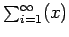to be nowhere differentiable for most any initial.

5. However, the particularmakes the proof easy, because the periodicity kills the higher terms of the series for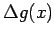, and usingfor the argument allows theth term to go to infinity and dominate the earlier terms of the series. It seems lucky in whatever sense luck exists in mathematics.